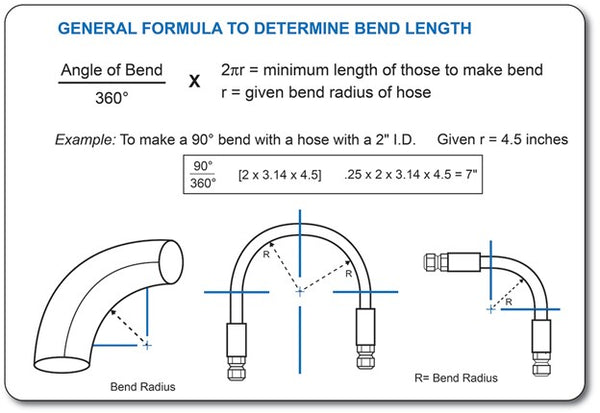# What is Hose Bend Radius & How to Use It

All hose spec sheets will list a bend radius for a given product. For example, a common 2” diameter rubber chemical hose may show a bend radius of 6”. The manufacturer has determined that this hose can be bent into a 6” radius to form a 90 degree or 180 degree turn without damaging the hose. When hoses are very short and turns are tight, the bend radius can be used to determine the minimum length of a given hose assembly.### LENGTH CALCULATION

Calculating the Hose Length

The formula for calculating the bent section of the hose length around a radius is derived from the basic formula that the circumference of a circle = 2πR, where R = the radius of the circle, and π = a constant, = 3.142.

So, if the hose goes around a 90˚ bend, which is 1/4 of a full circumference, and the radius of the bend is R, then the length of the hose around the bend is = 1/4 x 2πR. Or half way round, in a U-shape, = 1/2 x 2πR.

Note: In calculating the length of a hose assembly, the (non-flexible) length of the end fittings must be added in, also the length of any straight sections of hose.